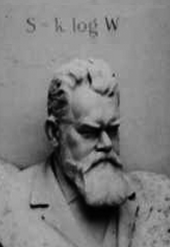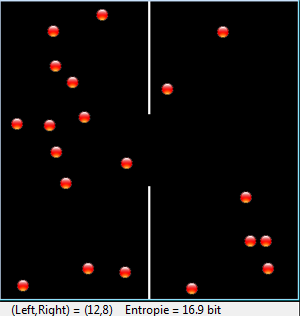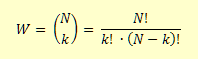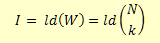deutsch     english    français     Print

## 10.7 INFORMATION & ORDER### INTRODUCTION

 Even though the word information is used often, it is not that easy to grasp the concept precisely and to make it measurable. In daily life, more information is associated with more knowledge and it is said that a person A informed in a certain thing knows more than an uninformed person B. In order to measure how much more information A has than B (or team B), or the lack of information of B with respect to A, you best imagine a TV quiz show. Person or team B has to find something out that only person A knows, for example their profession. Thereby B asks questions that A has to answer with a Yes or a No. The rules are as follows: The lack of information I of B in relation to A (in bits) is the number of questions with Yes/No answers that B (on average and at an optimal asking strategy) has to ask in order to have the same knowledge about a certain thing as A has. PROGRAMMING CONCEPTS: Information, information content, entropy

### NUMBER GUESSING GAME

You can enact such a guessing game in your classroom (or just in your head) as follows: Your classmate Judith is sent out of the room. One of the remaining W = 16 classmates receives a "desired" object, such as a bar of chocolate. After Judith is called back into the classroom, you and your classmates know who has the chocolate, but Judith does not. How big is her lack of information?

For the sake of simplicity, the classmates are numbered from 0 to 15 and Judith can now ask everyone questions in order to find out the secret number of the classmate with the chocolate. The less questions she asks, the better. One possibility would be for her to ask any random number: "Is it 13?". If the answer is No, she asks a different number.

 Judith could also proceed systematically and ask starting from 0: "Is it 0?", then "Is it 1?", etc. In your computer simulation, you determine how many questions she needs to ask on average (i.e. if the game is played many times) with this type of questions, in order to finally find out the secret number. Think about the following:If the secret number is 0, then 1 question is needed to find the answer; if the secret number is 1, 2 questions are needed, etc. If the secret number is 14, then 15 questions are needed, but when the secret number is 15, again only 15 questions are needed since it is the last possible option. The program plays the game 100,000 times and counts the number of questions it takes in each case until the secret number is determined. Those numbers are added up to finally determine the average.

```from random import randint

sum = 0
z = 100000
repeat z:
n = randint(0, 15)
if n != 15:
q = n + 1 # number of questions
else:
q = 15
sum += q
print "Mean:", sum / z
```
Highlight program code (Ctrl+C copy, Ctrl+V paste)

### MEMO

 As you can easily find out, with W = 32 numbers the information amounts to I = 5 bit, and with W = 64 numbers I = 6 bit. So apparently we get 2I = W or I = ld(W). It also does not need to be a number that one is searching for, but rather it can consist of any one state that has to be found out from W (equally probable) number of states. So the information at the knowledge of a state from W equally probable states is                                     I = ld(W)                (ld is the logarithm dualis, logarithm with base 2)

### INFORMATION CONTENT OF A WORD

Since the W states are equally likely, the probability of the states is p = 1/W. You can also write the information as follows:

I = ld(1/p) = -ld(p)

You certainly know that the probabilities of certain letters in a spoken language differ greatly. The following table shows the probabilities for the English language [more... The values depend on the type of text. The numbers correspond to a literary text].

 A 8.34% I 6.71% Q 0.09% Y 2.04% B 1.54% J 0.23% R 5.68% Z 0.96% C 2.73% K 0.87% S 6.11% D 4.14% L 4.24% T 9.37% E 12.60% M 2.53% U 2.85% F 2.03% N 6.80% V 1.06% G 1.92% O 7.70% W 2.34% H 6.11% P 1.66% X 0.20%

It is certainly not the case that the probability of a letter in a word is independent of the letters of the words you already know and the context in which the word appears. However, if we make this simple assumption, then the probability p of a 2-letter combination with the individual probabilities p1 and p2 is, according to the product rule, p = p1 * p2 and for the information:

I = -ld(p) = -ld(p1*p2 ) = -ld(p1 ) - ld(p2 )

or for arbitrarily many letters:

I = - ld(p1 ) - ld(p2 ) - ld(p3 ) - ... - ld(pn ) = - Σ ld(pi )

In your program, you can enter a word and you will get the information content of the word as an output. For this you should download the files with the letter frequencies in German, English, and French from here and copy them to the directory where your Python program is.

```from math import log

f = open("letterfreq_de.txt")
s = "{"
for line in f:
line = line[:-1] # remove trailing \n
s += line + ", "
f.close()
s = s[:-2]  # remove trailing ,
s += "}"
occurance = eval(s)

while True:
word = inputString("Enter a word")
I = 0
for letter in word.upper():
p = occurance[letter] / 100
I -= log(p, 2)
print word, "-> I =", round(I, 2), "bit"
```
Highlight program code (Ctrl+C copy, Ctrl+V paste)

### MEMO

 The data are saved in the text file line by line in the format 'letter' : percentage. In Python, a dictionary is well suited as a data structure for this. In order to convert the data information to a dictionary, the lines are read and packed into a string in standard dictionary format {key : value, key : value,...}. This string is interpreted as a line of code with eval() and a dictionary is created. As you can find out with your program, the information content of words with rare letters is greater. However, the information content determined this way has nothing to do with the importance or personal relevance of a certain word in a certain context, or in other words whether the information connected to the word is trivial for you or of crucial importance. It is far beyond the capabilities of today's information systems to determine a measure for this.

### EXERCISES

 1. When playing a guessing game with 16 classmates, the clever classmate could also ask something else by saying that you should imagine the secret number of the person with the chocolate as a binary number with 4 digits. Write out how you would ask this. 2. Use the list of English words from the file words-1\$.txt and determine the word with the smallest and largest information content from words that have a length of 5. Comment on your findings. Download the word lists from here. 3*. Determine the average number of questions it takes in the guessing of the number, in a situation where the numbers go from 0 to 15 and you use the questioning strategy "Is it 0, is it 1, etc." from a theoretical consideration..

### THE RELATIONSHIP BETWEEN DISORDER AND ENTROPY

There is an extremely interesting relationship between the information that we have about a system and the order of the system. For example, the order is certainly much greater when all students in a classroom are sitting at their desks, than when they are all moving around randomly in the room. In the disordered state, our lack of information of where each individual person is located is very high, thus we can use this lack of information as a measure of disorder. For this, one defines the concept of entropy:

 Entropy (in bits) is the lack of information I which we possess when describing the system macroscopically, in relation to someone who knows the microscopic state. For the entropy in (J/K) we set  S = k * ln2 * I The factor k is the Bolzmann constant. Entropy and the lack of information are thus the same except for one pre-factor. If we assume a system with W equally probable states, we have: S = k * ln2 * ldW or       S = k * log W This fundamental relationship comes from famous physicist Ludwig Boltzmann (1844-1900) and it is written on his gravestone.As you can determine, systems left to their own resources have the tendency to move from an ordered to a disordered state. For example:

• Passengers in a train spread out over the entire car
• Cigarette smoke spreads in a room
• A blot of ink disperses in a water glass
• The temperature between the coffee and the cup evens out over time
 Since the disordered state has a higher entropy than the ordered state, one can formulate this as a law of nature (2nd law of thermodynamics): In a closed system, the entropy either increases or remains the same, but it never decreases. You simulate a particle system with your program where the particles act as gas atoms and collide with each other while exchanging their direction and kinetic energy. All of the particles should first be located in the left part of the container, where they can escape through a hole in the divider. What happens?The module gamegrid is used for the animation. The particles are modeled in the class Particle that is derived from the class Actor. You can move the particles using the method act(), which is automatically called in each simulation cycle. The collisions are handled with collision events. For this, derive the class CollisionListener from GGActorCollisionListener and override the method collide(). This procedure is the same as what you saw in chapter 8.10 on Brownian Movement. The 20 particles are then divided into 4 different velocity groups. Since the velocities are switched between particles that collide, the total energy of the system remains constant, i.e. the system is closed.

```from gamegrid import *
from gpanel import *
from math import log

# =================== class Particle ====================
class Particle(Actor):
def __init__(self):
Actor.__init__(self, "sprites/ball_0.gif")

# Called when actor is added to gamegrid
def reset(self):
self.isLeft = True

pt = self.gameGrid.toPoint(self.getNextMoveLocation())
dir = self.getDirection()
# Left/right wall
if pt.x < 0 or pt.x > w:
self.setDirection(180 - dir)
# Top/bottom wall
if pt.y < 0 or pt.y > h:
self.setDirection(360 - dir)
# Separation
if (pt.y < h // 2 - r or pt.y > h // 2 + r) and \
pt.x > self.gameGrid.getPgWidth() // 2 - 2 and \
pt.x < self.gameGrid.getPgWidth() // 2 + 2:
self.setDirection(180 - dir)

self.move(distance)
if self.getX() < w // 2:
self.isLeft = True
else:
self.isLeft = False

def act(self):

def atLeft(self):
return self.isLeft

# =================== class CollisionListener =========
class CollisionListener(GGActorCollisionListener):
# Collision callback: just exchange direction and speed
def collide(self, a,  b):
dir1 = a.getDirection()
dir2 = b.getDirection()
sd1 = a.getSlowDown()
sd2 = b.getSlowDown()
a.setDirection(dir2)
a.setSlowDown(sd2)
b.setDirection(dir1)
b.setSlowDown(sd1)
return 5  # Wait a moment until collision is rearmed

# =================== Global sections =================
def drawSeparation():
getBg().setLineWidth(3)
getBg().drawLine(w // 2, 0, w // 2, h // 2 - r)
getBg().drawLine(w // 2, h, w // 2, h // 2 + r)

def init():
collisionListener = CollisionListener()
for i in range(nbParticles):
particles[i] = Particle()

# Put them at random locations, but apart of each other
ok = False
while not ok:
ok = True
loc = getRandomLocation()
if loc.x > w / 2 - 20:
ok = False
continue

for k in range(i):
dx = particles[k].getLocation().x - loc.x
dy = particles[k].getLocation().y - loc.y
if dx * dx + dy * dy < 300:
ok = False
delay(100)

# Select collision area
particles[i].setCollisionCircle(Point(0, 0), 8)
# Select collision listener

# Set speed in groups of 5
if i < 5:
particles[i].setSlowDown(2)
elif i < 10:
particles[i].setSlowDown(3)
elif i < 15:
particles[i].setSlowDown(4)

# Define collision partners
for i in range(nbParticles):
for k in range(i + 1, nbParticles):

def binomial(n, k):
if k < 0 or k > n:
return 0
if k == 0 or k == n:
return 1
k = min(k, n - k) # take advantage of symmetry
c = 1
for i in range(k):
c = c * (n - i) / (i + 1)
return c

r = 50  # Radius of hole
w = 400
h = 400
nbParticles = 20
particles =  * nbParticles
makeGPanel(Size(600, 300))
window(-6, 66, -2, 22)
title("Entropy")
windowPosition(600, 20)
drawGrid(0, 60, 0, 20)
makeGameGrid(w, h, 1, False)
setSimulationPeriod(10)
drawSeparation()
setTitle("Entropy")
show()
init()
doRun()

t = 0
while not isDisposed():
nbLeft = 0
for particle in particles:
if particle.atLeft():
nbLeft += 1
entropy = round(log(binomial(nbParticles, nbLeft), 2), 1)
setStatusText("(Left,Right) = (" + str(nbLeft) + "," +
str(nbParticles-nbLeft)+ ")"+" Entropie = "+str(entropy)+" bit")
if t % 60 == 0:
clear()
lineWidth(1)
drawGrid(0, 60, 0, 20)
lineWidth(3)
move(0, entropy)
else:
draw(t % 60, entropy)
t += 1
delay(1000)
dispose() # GPanel
```
Highlight program code (Ctrl+C copy, Ctrl+V paste)

### MEMO

When seen from the outside (macroscopically), a state is determined by the number k of particles in the right part, and thus N - k in the left part. A macroscopic observation lacks the precise knowledge, which k of the N numbered particles are on the right. According to combinatorics there aresuch possibilities. The lack of information is thereforeand the entropyThe temporal process is plotted in a GPanel graphic. You can clearly see that the particles distribute themselves throughout the entire container over time, but there is also a chance that all particles end up again in the left half. The probability of this decreases rapidly with an increasing number of particles. The 2nd law thus relies on a statistical property of multiple-particle systems.

Since the reverse process is not observed, these processes are called irreversible. However, there are indeed processes that passed from disorder to order. For this, they need an "ordering constraint" [more... This is known as a "enslavement"].

Some examples are:

• The matter of the cosmos forms galaxies, stars, and planetary systems
• Life emerges from non-living matter
• With sufficient enforcement the disorder in kitchens, or classrooms, diminishes
• Clouds (liquid) and ice (solid) emerge from the cooling of water vapor (gas). Thus, phase transitions change the order
• Concert-goers flock back to their seats after the break

These processes decrease the disorder, and therefore the entropy, so that structures gradually become visible from chaos.

### EXERCISES

 1 Modify the gas simulation so that after 50 seconds a “demon” makes sure that the particles only fly from right to left through the gap, but not from left to right. Show that entropy now decreases. 2 Find other examples of systems that: a. switch from order to disorder b. switch from disorder to order. State the ordering constraint.